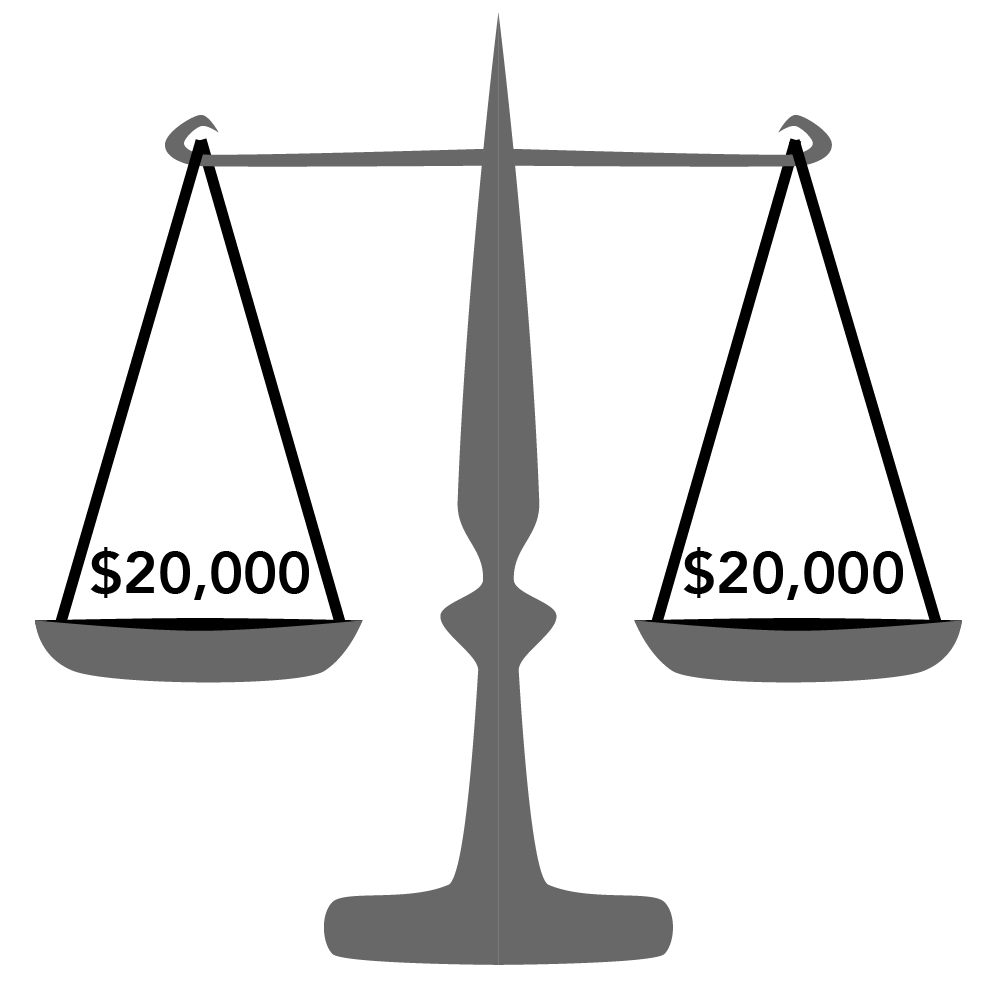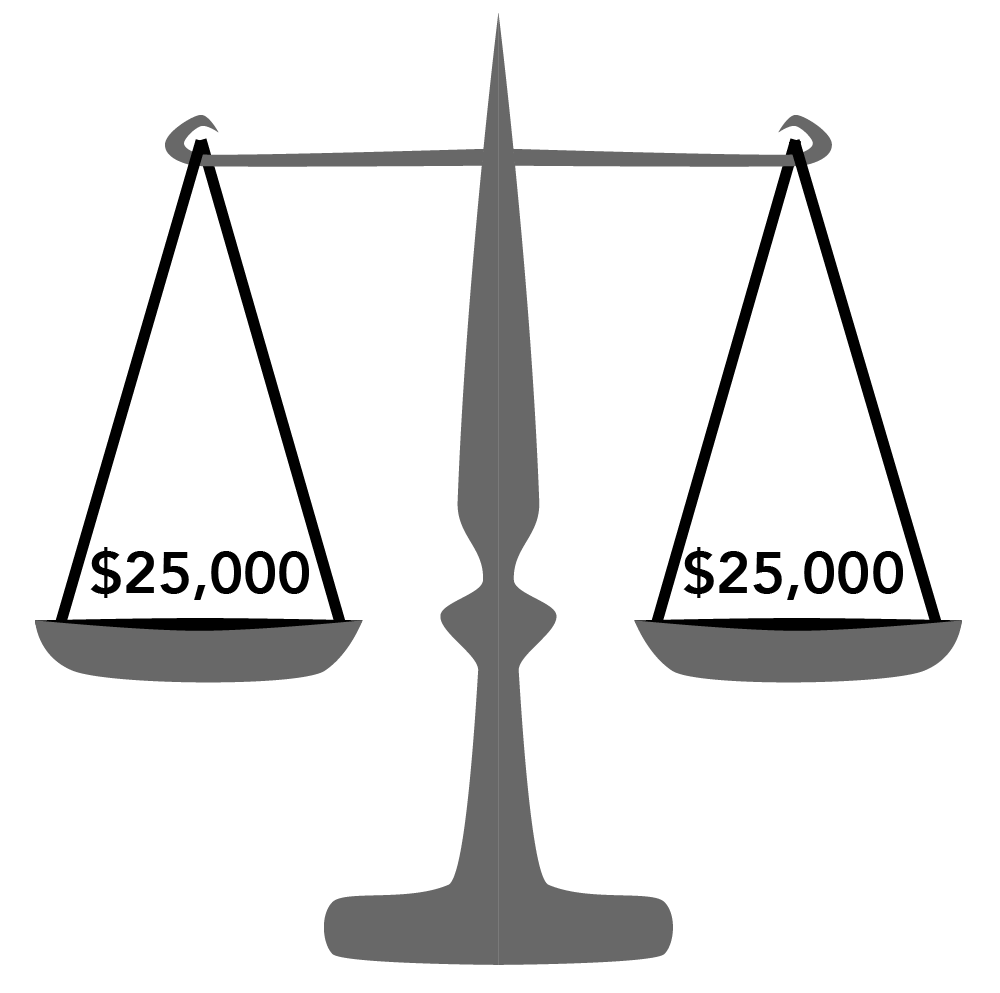## The Accounting Equation

### Learning Outcomes

• Explain the basic accounting equation

One of the cornerstones of financial accounting is the accounting equation, which in its simplest form, looks like this:

$\text{A}=\text{L}+\text{OE}$

This equation has to always stay in balance.

An owner registers their new company with the state department of business licensing. They take their business license down to the bank and transfer $20,000 of their own money into a new business account. They have now “capitalized” their business, which means they made a contribution to capital, which increases owner’s equity. At the same time, they have increased the balance in their checking account. From a bookkeeping perspective, you have to make two entries for this one business transaction, and these two entries balance each other out. $\text{A}=\text{L}+\text{OE}$ $\20,000=\0+\20,000$Now, suppose the owner also borrows$5,000 from the bank, which is then deposited into their account. A loan will not increase their equity. It is not a capital contribution. It is debt, which is a liability.

Now, the equation looks like this:

$\text{A}=\text{L}+\text{OE}$

$\25,000\left(\text{cash}\right)=\5,000\left(\text{bank loan}\right)+\20,000\left(\text{original capital contribution}\right)$Assets are what the business owns. Liabilities are what it owes, and equity is the amount of the company that belongs to the business owner.

### Balance in a Home Loan

Think of the equation in terms of a house. Say the house costs $250,000 and you owe$200,000 to the bank. Your equity in the home is \$50,000.

$\text{A}=\text{L}+\text{OE}$

can also be stated as:

$\text{A}-\text{L}=\text{OE}$

$\text{Assets}-\text{Liabilities}=\text{Owner's Equity}$

In the case of the house cited above, the equation is $\250,000 - \200,000 = \50,000$.

Commit this important accounting concept to memory: Assets = Liabilities + Owner’s Equity